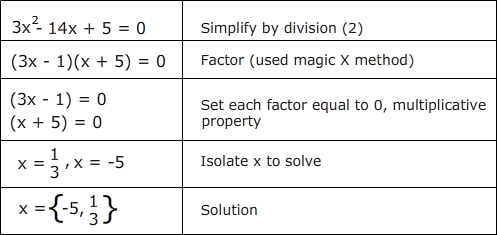Quiz
* Description/Instructions
A composite expression is a product of two or more expressions. Factoring breaks apart the expression into manageable multipliers in order to easily find the zeros of the composite expression. Factoring is an important process in algebra to simplify expressions, simplify fractions, and solve equations. Use any method of factoring to solve the following quadratic equations below.

For example: 6x2 - 28x + 10 = 0Group: Algebra Algebra Quizzes